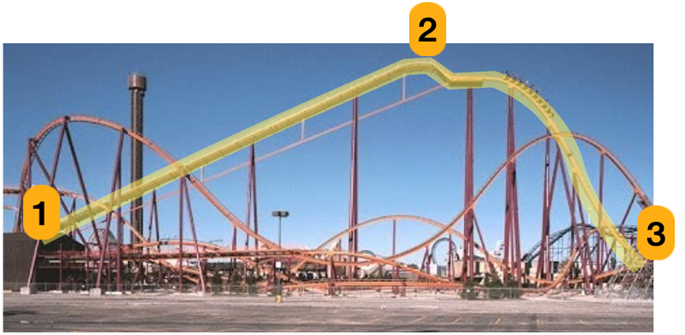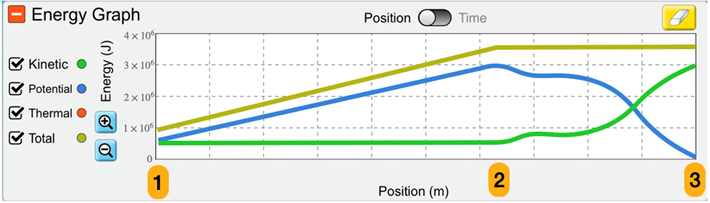# Question The track is highlighted in yellow for our analysis. From point 1 to point 2, the cars are being pulled upward at a constant low speed. From point 2 to point 3 the carts are rolling down the track under the influence of gravity. Assume friction is negligible. A PhET style energy graph (with 1 intentional mistake) appears below: How can the total energy be increasing from point 1 to point 2? Where is that energy coming from? How come the Total Energy is greater than the potential energy from point 1 to point 2? How come the Kinetic Energy is constant between points 1 and 2? How come the total energy stays constant between point 2 and 3? At what point in the graph do you notice there is a mistake? Look for something that is physically impossible, describe what you see and explain how you know that it is wrong. Please provide explanations for each question. 2 1 3 Energy Graph Position Time 4 x 10 3 x 10 Kinetic Potential Thermal Total O Energy (J) 2 x 10 1 x 10 0 1 2 3 Position (m)The track is highlighted in yellow for our analysis. From point 1 to point 2, the cars are being pulled upward at a constant low speed. From point 2 to point 3 the carts are rolling down the track under the influence of gravity. Assume friction is negligible. A PhET style energy graph (with 1 intentional mistake) appears below:

1. How can the total energy be increasing from point 1 to point 2? Where is that energy coming from?
2. How come the Total Energy is greater than the potential energy from point 1 to point 2?
3. How come the Kinetic Energy is constant between points 1 and 2?
4. How come the total energy stays constant between point 2 and 3?
5. At what point in the graph do you notice there is a mistake? Look for something that is physically impossible, describe what you see and explain how you know that it is wrong.Please provide explanations for each question.

Transcribed Image Text: 2 1 3 Energy Graph Position Time 4 x 10 3 x 10 Kinetic Potential Thermal Total O Energy (J) 2 x 10 1 x 10 0 1 2 3 Position (m)
More
Transcribed Image Text: 2 1 3 Energy Graph Position Time 4 x 10 3 x 10 Kinetic Potential Thermal Total O Energy (J) 2 x 10 1 x 10 0 1 2 3 Position (m)